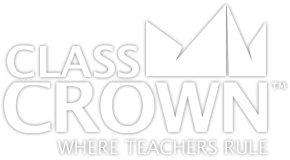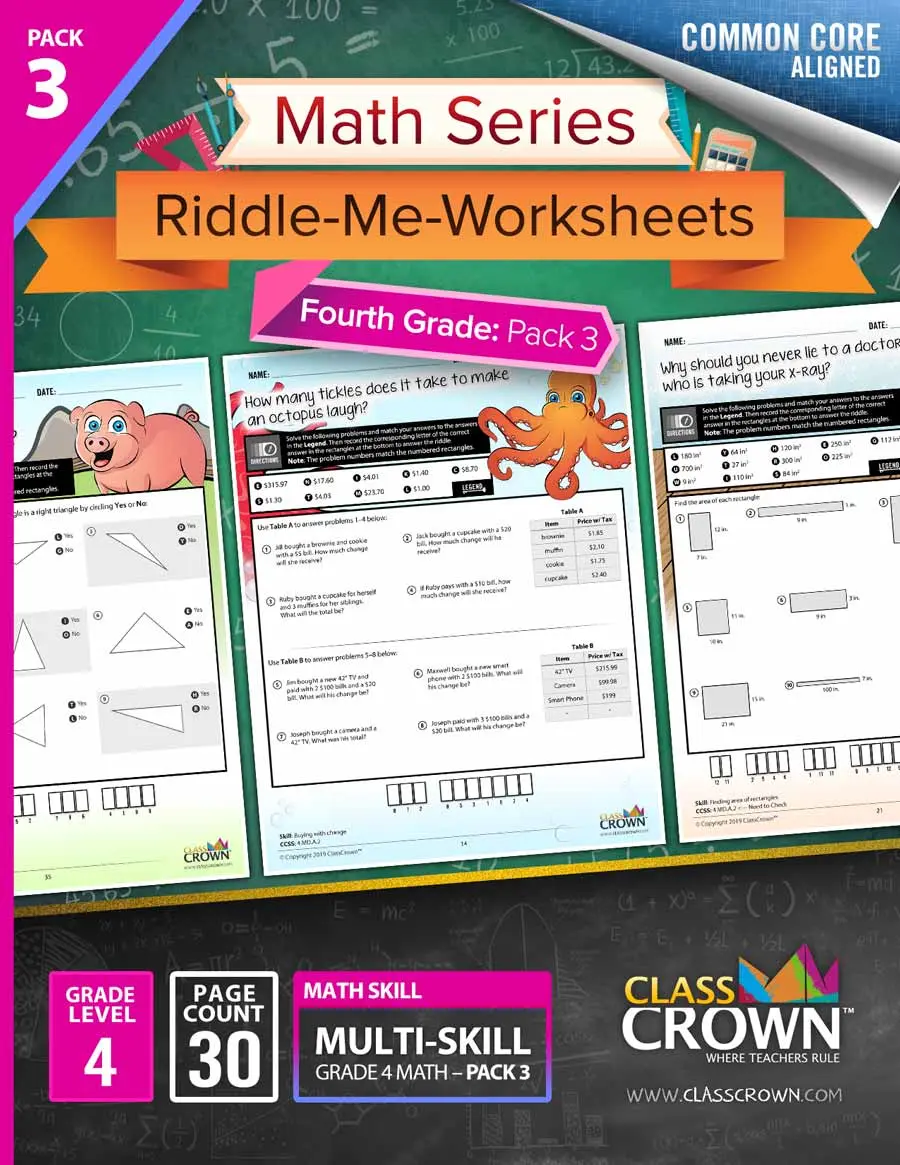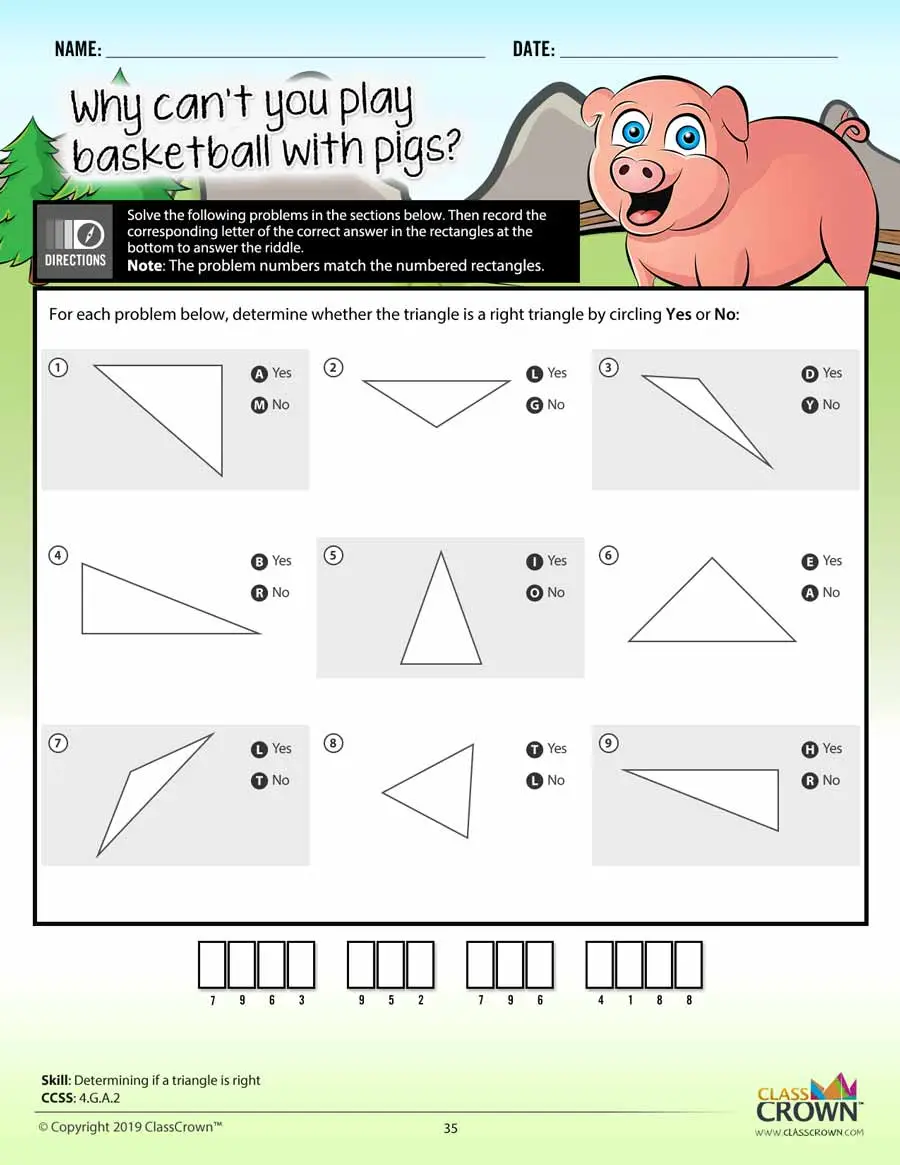Math Worksheets

# 4th Grade Math Worksheets: Pack 3

## Self-checking math worksheets with riddles to keep kids motivated.

Rating: 4.7/5
(0 Reviews)
4th
WORKSHEET COUNT
30
FORMAT(S)
PDF
Yes
\$8.99
This comprehensive bundle includes 4th grade packs 1–3.
\$20.99
\$26.97
Save 22%
The Details
Description
• Do your students ever complete an entire math worksheet, only to find that they have done most of the problems incorrectly and clearly have a misconception?
• ClassCrown’s Riddle-Me-Worksheets help to prevent this problem by introducing a puzzle aspect to math, giving students immediate feedback as to whether or not they are solving problems correctly. If the answer to the riddle isn't correct, the student knows which problems he's made an error on.
Specifics
• Subject:  Volume, Area, Geometry, +more!
• Product Count:  30
• Full Page Count:  52
• File Format(s):  PDF

What's Included?
• Estimating capacity, American
• Estimating length, American
• Conversion tables
• Estimating length, metric
• Measuring in centimeters
• Estimating weight, American
• Comparing weight, metric
• Determining change, multiple costs
• Determining change, one cost, multiple items
• Solving word problems involving distance
• Solving word problems involving intervals of time
• Solving problems involving liquid volumes
• Solving word problems involving mass
• Finding area of rectangles
• Finding perimeter of rectangles
• Finding area of rectangles, word problems
• Finding perimeter, word problems
• Interpreting line plots that include fractions
• Interpreting line plots that include fractions
• Estimating angle measurement
• Determining if an angle is acute, obtuse, right, or straight
• Determining angles using a protractor
• Determining value of unknown angles
• Comparing angles to 90 degrees
• Determining if an angle is acute, obtuse, right, or straight
• Finding angles within shapes
• Determining parallel, perpendicular, or intersecting lines
• Determining if a triangle is right
• Determining symmetry
CCSS: : 4.MD.A.1, 4.MD.A.2, 4.MD.A.3, 4.MD.B.4, 4.MD.C.5.A, 4.MD.C.6, 4.MD.C.7, 4.G.A.1, 4.G.A.2, 4.G.A.3
Reviews
Overall Rating: 4.7/5
(Showing 0 of 0)
See More Reviews @ TpTSelf Checking

Our math worksheets introduce a puzzle aspect to math, giving students immediate feedback as to whether or not they are solving problems correctly. If the answer to the riddle isn't spelled correctly, the student knows which problems he's made an error on.Fun Puzzle AspectImmediate Feedback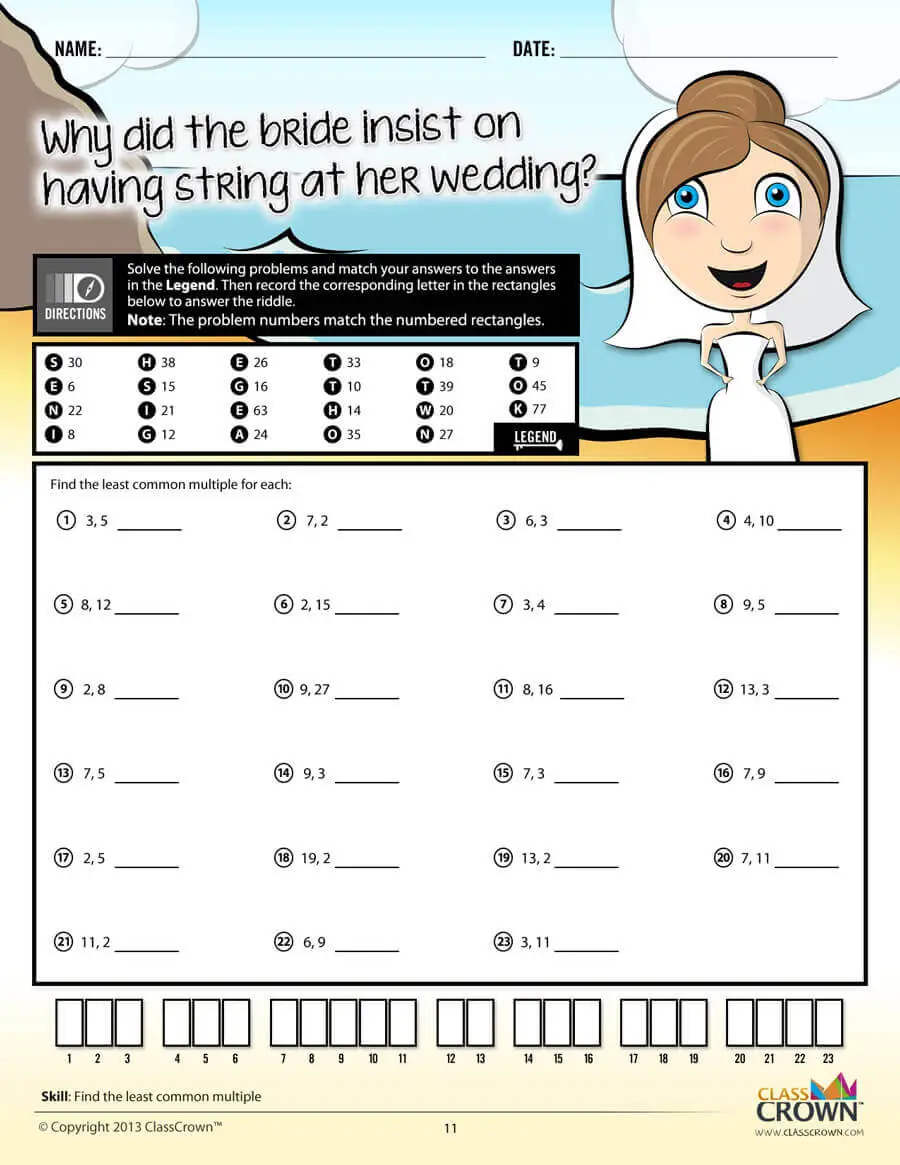Problem Solving Motivation

Each math worksheet contains a riddle that the student solves by completeing all the problems on the worksheet. This keeps kids motivated to complete each problem so that they can find the answer to the riddle.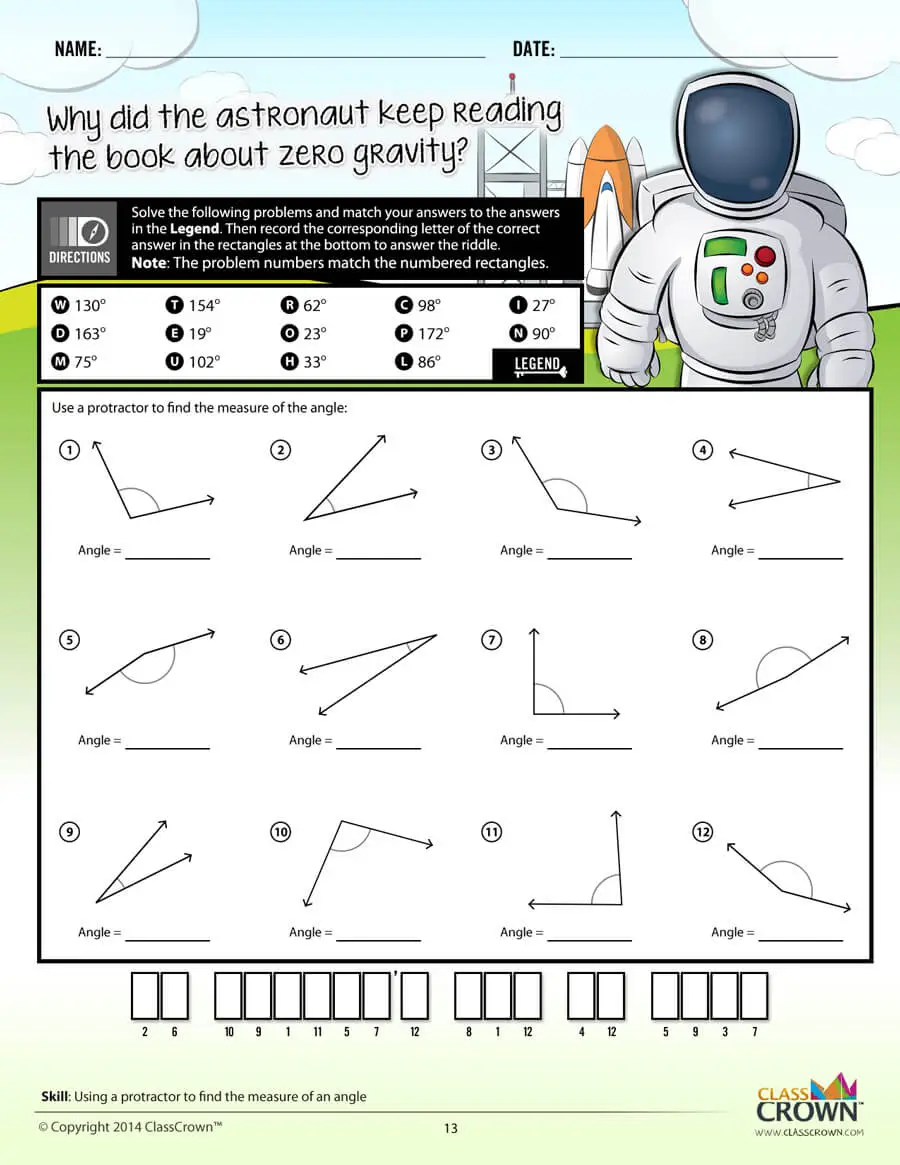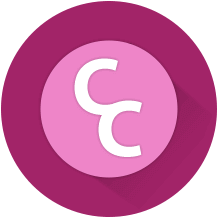Common Core Aligned

All our math worksheet packs are designed with Common Core in mind. That way you don’t have to worry about whether your math ciriculum is aligned or not when you incorpoate ClassCrown Riddle-Me-Worksheets in your lesson plans.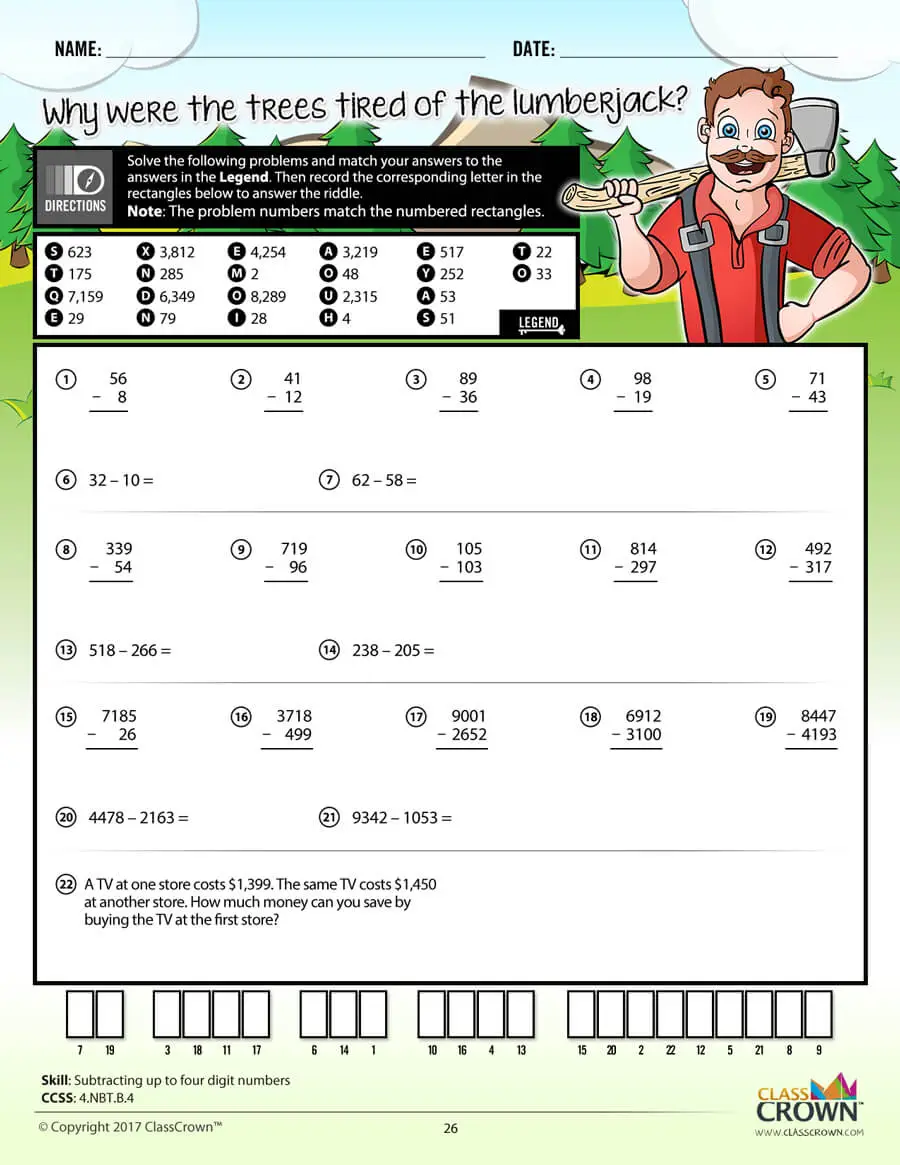High Quality Design

Each page of our math worksheets has been produced in high resolution at 144 dpi and designed in full, vibrant color for maximum quality. They look stunning whether you are printing in color or black and white.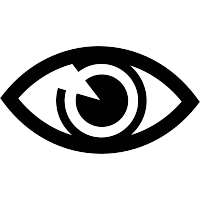High Resolution
(144 dpi)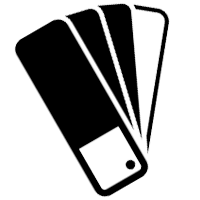Stunning Color & Clarity